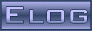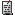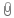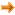Back Midas Rome Roody Rootana
 Midas DAQ System Not logged in18 Dec 2020, Stefan Ritt, Suggestion, Code formatting04 Jan 2021, Stefan Ritt, Suggestion, Code formattingMessage ID: 2062     Entry time: 18 Dec 2020     Reply to this: 2063
 Author: Stefan Ritt Topic: Suggestion Subject: Code formatting
```May I ask for your quick opinion on code formatting. MIDAS had a coding style
which pretty much followed the ROOT coding style described at

https://root.cern/contribute/coding_conventions/

so we followed the "3 spaces indent" convention, braces according to Kernigham &
Ritchie and a few other things. I see however that code written by different
people still is formatted differently, like spaces before and after comparators
etc. I wonder if it would make sense to keep a consistent code formatting through
the whole midas repository.

Looking again at what the ROOT guys doe (see link above), they have a ClangFormat
file, which I attached to this post. Putting this file into the root of midas
ensures that all files are formatted in exactly the same way, which would increase

The nice thing with ClangFormat is that can be integrated into my editor (Clion) as
well as in emacs and vim:

https://clang.llvm.org/docs/ClangFormat.html

This would also make the emacs settings in our files obsolete:

/* emacs
* Local Variables:
* tab-width: 8
* c-basic-offset: 3
* indent-tabs-mode: nil
* End:
*/

I don't like these because they are only for people using emacs. If everybody would
put statements into the files with their favourite editor, all our source files
would be cluttered quite a bit.

So the question is now how style to use? I attached different trials with a simple
file from the distribution, so you can see the differences. They use the style from

- LLVM
- ROOT
- GNU

I consciously skipped the "Microsoft" style ;-)

Which one should we settle on? Any opinion? If I don't hear anything, I will pick a
style at the end of this year 2020. I have a slight favour of the ROOT style, although
I don't like that the "case" is not indented there under the opening brace of the
switch statement which seems inconsistent to me. The only one doing that right is the
Google format, but that one has an indentation of 2 chars instead our usual 3 chars.
At the end of the day I think it's not so important on which style we agree, as long
as we DO have a common style for all midas files.

Best,
Stefan```
 Attachment 1: .clang-format  2 kB
```---
Language:        Cpp
# BasedOnStyle:  LLVM
AccessModifierOffset: -3
AlignAfterOpenBracket: Align
AlignConsecutiveAssignments: true
# This would be nice to have but seems to also (mis)align function parameters
AlignConsecutiveDeclarations: true
AlignEscapedNewlinesLeft: true
AlignOperands:   true
AllowAllParametersOfDeclarationOnNextLine: true
AllowShortBlocksOnASingleLine: false
AllowShortCaseLabelsOnASingleLine: true
AllowShortFunctionsOnASingleLine: Inline
AllowShortIfStatementsOnASingleLine: true
AllowShortLoopsOnASingleLine: true
# This option is "deprecated and is retained for backwards compatibility."
# AlwaysBreakAfterDefinitionReturnType: None
AlwaysBreakAfterReturnType: None
AlwaysBreakBeforeMultilineStrings: false
AlwaysBreakTemplateDeclarations: true
BinPackArguments: true
BinPackParameters: true
BraceWrapping:
AfterClass:      false
AfterControlStatement: false
AfterEnum:       false
AfterFunction:   true
AfterNamespace:  false
AfterObjCDeclaration: false
AfterStruct:     false
AfterUnion:      false
BeforeCatch:     false
BeforeElse:      false
IndentBraces:    false
BreakBeforeBinaryOperators: None
BreakBeforeBraces: Custom
BreakBeforeTernaryOperators: true
BreakConstructorInitializersBeforeComma: false
ColumnLimit:     120
CommentPragmas:  '^ IWYU pragma:'
ConstructorInitializerAllOnOneLineOrOnePerLine: false
ConstructorInitializerIndentWidth: 3
ContinuationIndentWidth: 3
Cpp11BracedListStyle: true
DerivePointerAlignment: false
DisableFormat:   false
ExperimentalAutoDetectBinPacking: false
ForEachMacros:   [ foreach, Q_FOREACH, BOOST_FOREACH ]
IncludeCategories:
- Regex:           '^("|<)T'
Priority:        4
- Regex:           '^("|<)ROOT/'
Priority:        5
- Regex:           '^<.*\.h>'
Priority:        1
- Regex:           '^"(llvm|llvm-c|clang|clang-c)/'
Priority:        2
- Regex:           '^(<|"(gtest|isl|json)/)'
Priority:        3
- Regex:           '.*'
Priority:        6
IndentCaseLabels: false
IndentWidth:     3
IndentWrappedFunctionNames: false
KeepEmptyLinesAtTheStartOfBlocks: true
MacroBlockBegin: ''
MacroBlockEnd:   ''
MaxEmptyLinesToKeep: 1
NamespaceIndentation: None
ObjCBlockIndentWidth: 3
ObjCSpaceAfterProperty: false
ObjCSpaceBeforeProtocolList: true
PenaltyBreakBeforeFirstCallParameter: 19
PenaltyBreakComment: 300
PenaltyBreakFirstLessLess: 120
PenaltyBreakString: 1000
PenaltyExcessCharacter: 1000000
PenaltyReturnTypeOnItsOwnLine: 60000
PointerAlignment: Right
SortIncludes: false
SpaceAfterCStyleCast: false
SpaceBeforeAssignmentOperators: true
SpaceBeforeParens: ControlStatements
SpaceInEmptyParentheses: false
SpacesInAngles:  false
SpacesInContainerLiterals: true
SpacesInCStyleCastParentheses: false
SpacesInParentheses: false
SpacesInSquareBrackets: false
Standard:        Cpp11
TabWidth:        3
UseTab:          Never
...
```
 Attachment 2: cnaf_callback_llvm.cxx  3 kB
```/********************************************************************\

Name:         cnaf_callback.c
Created by:   Stefan Ritt
Created by:   moved here from mfe.c by Konstantin Olchanski

Contents:     The system part of the MIDAS frontend. Has to be
linked with user code to form a complete frontend

\$Id\$

\********************************************************************/

#include <stdio.h>
#include <assert.h>
#include "midas.h"
#include "msystem.h"
#include "mcstd.h"

/*------------------------------------------------------------------*/

static int cnaf_debug = 0;

INT cnaf_callback(INT index, void *prpc_param[]) {
DWORD cmd, b, c, n, a, f, *pdword, *size, *x, *q, dtemp;
WORD *pword, *pdata, temp;
INT i, count;

/* Decode parameters */
cmd = CDWORD(0);
b = CDWORD(1);
c = CDWORD(2);
n = CDWORD(3);
a = CDWORD(4);
f = CDWORD(5);
pdword = CPDWORD(6);
pword = CPWORD(6);
pdata = CPWORD(6);
size = CPDWORD(7);
x = CPDWORD(8);
q = CPDWORD(9);

/* determine repeat count */
if (index == RPC_CNAF16)
count = *size / sizeof(WORD); /* 16 bit */
else
count = *size / sizeof(DWORD); /* 24 bit */

switch (cmd) {
/*---- special commands ----*/

case CNAF_INHIBIT_SET: cam_inhibit_set(c);
break;
case CNAF_INHIBIT_CLEAR: cam_inhibit_clear(c);
break;
case CNAF_CRATE_CLEAR: cam_crate_clear(c);
break;
case CNAF_CRATE_ZINIT: cam_crate_zinit(c);
break;

case CNAF_TEST: break;

case CNAF:
if (index == RPC_CNAF16) {
for (i = 0; i < count; i++)
if (f < 16)
cam16i_q(c, n, a, f, pword++, (int *) x, (int *) q);
else if (f < 24)
cam16o_q(c, n, a, f, pword[i], (int *) x, (int *) q);
else
cam16i_q(c, n, a, f, &temp, (int *) x, (int *) q);
} else {
for (i = 0; i < count; i++)
if (f < 16)
cam24i_q(c, n, a, f, pdword++, (int *) x, (int *) q);
else if (f < 24)
cam24o_q(c, n, a, f, pdword[i], (int *) x, (int *) q);
else
cam24i_q(c, n, a, f, &dtemp, (int *) x, (int *) q);
}

break;

case CNAF_nQ:
if (index == RPC_CNAF16) {
if (f < 16) {
cam16i_rq(c, n, a, f, &pword, count);
*size = (POINTER_T) pword - (POINTER_T) pdata;
}
} else {
if (f < 16) {
cam24i_rq(c, n, a, f, &pdword, count);
*size = (POINTER_T) pdword - (POINTER_T) pdata;
}
}

/* return reduced return size */
break;

default: printf("cnaf: Unknown command 0x%X\n", (unsigned int) cmd);
}

if (cnaf_debug) {
if (index == RPC_CNAF16)
printf("cmd=%d r=%d c=%d n=%d a=%d f=%d d=%X x=%d q=%d\n", (int) cmd, (int) count, (int) c, (int) n, (int) a,
(int) f, (int) pword, (int) *x, (int) *q);
else if (index == RPC_CNAF24)
printf("cmd=%d r=%d c=%d n=%d a=%d f=%d d=%X x=%d q=%d\n", (int) cmd, (int) count, (int) c, (int) n, (int) a,
(int) f, (int) pdword, (int) *x, (int) *q);
}

return RPC_SUCCESS;
}

void register_cnaf_callback(int debug) {
cnaf_debug = debug;
/* register CNAF callback */
cm_register_function(RPC_CNAF16, cnaf_callback);
cm_register_function(RPC_CNAF24, cnaf_callback);
}

/* end file */
```
 Attachment 3: cnaf_callback_root.cxx  3 kB
```/********************************************************************\

Name:         cnaf_callback.c
Created by:   Stefan Ritt
Created by:   moved here from mfe.c by Konstantin Olchanski

Contents:     The system part of the MIDAS frontend. Has to be
linked with user code to form a complete frontend

\$Id\$

\********************************************************************/

#include <stdio.h>
#include <assert.h>
#include "midas.h"
#include "msystem.h"
#include "mcstd.h"

/*------------------------------------------------------------------*/

static int cnaf_debug = 0;

INT cnaf_callback(INT index, void *prpc_param[])
{
DWORD cmd, b, c, n, a, f, *pdword, *size, *x, *q, dtemp;
WORD *pword, *pdata, temp;
INT   i, count;

/* Decode parameters */
cmd    = CDWORD(0);
b      = CDWORD(1);
c      = CDWORD(2);
n      = CDWORD(3);
a      = CDWORD(4);
f      = CDWORD(5);
pdword = CPDWORD(6);
pword  = CPWORD(6);
pdata  = CPWORD(6);
size   = CPDWORD(7);
x      = CPDWORD(8);
q      = CPDWORD(9);

/* determine repeat count */
if (index == RPC_CNAF16)
count = *size / sizeof(WORD); /* 16 bit */
else
count = *size / sizeof(DWORD); /* 24 bit */

switch (cmd) {
/*---- special commands ----*/

case CNAF_INHIBIT_SET: cam_inhibit_set(c); break;
case CNAF_INHIBIT_CLEAR: cam_inhibit_clear(c); break;
case CNAF_CRATE_CLEAR: cam_crate_clear(c); break;
case CNAF_CRATE_ZINIT: cam_crate_zinit(c); break;

case CNAF_TEST: break;

case CNAF:
if (index == RPC_CNAF16) {
for (i = 0; i < count; i++)
if (f < 16)
cam16i_q(c, n, a, f, pword++, (int *)x, (int *)q);
else if (f < 24)
cam16o_q(c, n, a, f, pword[i], (int *)x, (int *)q);
else
cam16i_q(c, n, a, f, &temp, (int *)x, (int *)q);
} else {
for (i = 0; i < count; i++)
if (f < 16)
cam24i_q(c, n, a, f, pdword++, (int *)x, (int *)q);
else if (f < 24)
cam24o_q(c, n, a, f, pdword[i], (int *)x, (int *)q);
else
cam24i_q(c, n, a, f, &dtemp, (int *)x, (int *)q);
}

break;

case CNAF_nQ:
if (index == RPC_CNAF16) {
if (f < 16) {
cam16i_rq(c, n, a, f, &pword, count);
*size = (POINTER_T)pword - (POINTER_T)pdata;
}
} else {
if (f < 16) {
cam24i_rq(c, n, a, f, &pdword, count);
*size = (POINTER_T)pdword - (POINTER_T)pdata;
}
}

/* return reduced return size */
break;

default: printf("cnaf: Unknown command 0x%X\n", (unsigned int)cmd);
}

if (cnaf_debug) {
if (index == RPC_CNAF16)
printf("cmd=%d r=%d c=%d n=%d a=%d f=%d d=%X x=%d q=%d\n", (int)cmd, (int)count, (int)c, (int)n, (int)a,
(int)f, (int)pword, (int)*x, (int)*q);
else if (index == RPC_CNAF24)
printf("cmd=%d r=%d c=%d n=%d a=%d f=%d d=%X x=%d q=%d\n", (int)cmd, (int)count, (int)c, (int)n, (int)a,
(int)f, (int)pdword, (int)*x, (int)*q);
}

return RPC_SUCCESS;
}

void register_cnaf_callback(int debug)
{
cnaf_debug = debug;
/* register CNAF callback */
cm_register_function(RPC_CNAF16, cnaf_callback);
cm_register_function(RPC_CNAF24, cnaf_callback);
}

/* end file */
```
 Attachment 4: cnaf_callback_gnu.cxx  3 kB
```/********************************************************************\

Name:         cnaf_callback.c
Created by:   Stefan Ritt
Created by:   moved here from mfe.c by Konstantin Olchanski

Contents:     The system part of the MIDAS frontend. Has to be
linked with user code to form a complete frontend

\$Id\$

\********************************************************************/

#include <stdio.h>
#include <assert.h>
#include "midas.h"
#include "msystem.h"
#include "mcstd.h"

/*------------------------------------------------------------------*/

static int cnaf_debug = 0;

INT cnaf_callback (INT index, void *prpc_param[])
{
DWORD cmd, b, c, n, a, f, *pdword, *size, *x, *q, dtemp;
WORD *pword, *pdata, temp;
INT i, count;

/* Decode parameters */
cmd = CDWORD(0);
b = CDWORD(1);
c = CDWORD(2);
n = CDWORD(3);
a = CDWORD(4);
f = CDWORD(5);
pdword = CPDWORD(6);
pword = CPWORD(6);
pdata = CPWORD(6);
size = CPDWORD(7);
x = CPDWORD(8);
q = CPDWORD(9);

/* determine repeat count */
if (index == RPC_CNAF16)
count = *size / sizeof (WORD); /* 16 bit */
else
count = *size / sizeof (DWORD); /* 24 bit */

switch (cmd)
{
/*---- special commands ----*/

case CNAF_INHIBIT_SET: cam_inhibit_set (c);
break;
case CNAF_INHIBIT_CLEAR: cam_inhibit_clear (c);
break;
case CNAF_CRATE_CLEAR: cam_crate_clear (c);
break;
case CNAF_CRATE_ZINIT: cam_crate_zinit (c);
break;

case CNAF_TEST: break;

case CNAF:
if (index == RPC_CNAF16)
{
for (i = 0; i < count; i++)
if (f < 16)
cam16i_q (c, n, a, f, pword++, (int *) x, (int *) q);
else if (f < 24)
cam16o_q (c, n, a, f, pword[i], (int *) x, (int *) q);
else
cam16i_q (c, n, a, f, &temp, (int *) x, (int *) q);
}
else
{
for (i = 0; i < count; i++)
if (f < 16)
cam24i_q (c, n, a, f, pdword++, (int *) x, (int *) q);
else if (f < 24)
cam24o_q (c, n, a, f, pdword[i], (int *) x, (int *) q);
else
cam24i_q (c, n, a, f, &dtemp, (int *) x, (int *) q);
}

break;

case CNAF_nQ:
if (index == RPC_CNAF16)
{
if (f < 16)
{
cam16i_rq (c, n, a, f, &pword, count);
*size = (POINTER_T) pword - (POINTER_T) pdata;
}
}
else
{
if (f < 16)
{
cam24i_rq (c, n, a, f, &pdword, count);
*size = (POINTER_T) pdword - (POINTER_T) pdata;
}
}

/* return reduced return size */
break;

default: printf ("cnaf: Unknown command 0x%X\n", (unsigned int) cmd);
}

if (cnaf_debug)
{
if (index == RPC_CNAF16)
printf ("cmd=%d r=%d c=%d n=%d a=%d f=%d d=%X x=%d q=%d\n", (int) cmd, (int) count, (int) c, (int) n, (int) a,
(int) f, (int) pword, (int) *x, (int) *q);
else if (index == RPC_CNAF24)
printf ("cmd=%d r=%d c=%d n=%d a=%d f=%d d=%X x=%d q=%d\n", (int) cmd, (int) count, (int) c, (int) n, (int) a,
(int) f, (int) pdword, (int) *x, (int) *q);
}

return RPC_SUCCESS;
}

void register_cnaf_callback (int debug)
{
cnaf_debug = debug;
/* register CNAF callback */
cm_register_function (RPC_CNAF16, cnaf_callback);
cm_register_function (RPC_CNAF24, cnaf_callback);
}

/* end file */
```
```/********************************************************************\

Name:         cnaf_callback.c
Created by:   Stefan Ritt
Created by:   moved here from mfe.c by Konstantin Olchanski

Contents:     The system part of the MIDAS frontend. Has to be
linked with user code to form a complete frontend

\$Id\$

\********************************************************************/

#include <stdio.h>
#include <assert.h>
#include "midas.h"
#include "msystem.h"
#include "mcstd.h"

/*------------------------------------------------------------------*/

static int cnaf_debug = 0;

INT cnaf_callback(INT index, void *prpc_param[]) {
DWORD cmd, b, c, n, a, f, *pdword, *size, *x, *q, dtemp;
WORD *pword, *pdata, temp;
INT i, count;

/* Decode parameters */
cmd = CDWORD(0);
b = CDWORD(1);
c = CDWORD(2);
n = CDWORD(3);
a = CDWORD(4);
f = CDWORD(5);
pdword = CPDWORD(6);
pword = CPWORD(6);
pdata = CPWORD(6);
size = CPDWORD(7);
x = CPDWORD(8);
q = CPDWORD(9);

/* determine repeat count */
if (index == RPC_CNAF16)
count = *size / sizeof(WORD); /* 16 bit */
else
count = *size / sizeof(DWORD); /* 24 bit */

switch (cmd) {
/*---- special commands ----*/

case CNAF_INHIBIT_SET: cam_inhibit_set(c);
break;
case CNAF_INHIBIT_CLEAR: cam_inhibit_clear(c);
break;
case CNAF_CRATE_CLEAR: cam_crate_clear(c);
break;
case CNAF_CRATE_ZINIT: cam_crate_zinit(c);
break;

case CNAF_TEST: break;

case CNAF:
if (index == RPC_CNAF16) {
for (i = 0; i < count; i++)
if (f < 16)
cam16i_q(c, n, a, f, pword++, (int *) x, (int *) q);
else if (f < 24)
cam16o_q(c, n, a, f, pword[i], (int *) x, (int *) q);
else
cam16i_q(c, n, a, f, &temp, (int *) x, (int *) q);
} else {
for (i = 0; i < count; i++)
if (f < 16)
cam24i_q(c, n, a, f, pdword++, (int *) x, (int *) q);
else if (f < 24)
cam24o_q(c, n, a, f, pdword[i], (int *) x, (int *) q);
else
cam24i_q(c, n, a, f, &dtemp, (int *) x, (int *) q);
}

break;

case CNAF_nQ:
if (index == RPC_CNAF16) {
if (f < 16) {
cam16i_rq(c, n, a, f, &pword, count);
*size = (POINTER_T) pword - (POINTER_T) pdata;
}
} else {
if (f < 16) {
cam24i_rq(c, n, a, f, &pdword, count);
*size = (POINTER_T) pdword - (POINTER_T) pdata;
}
}

/* return reduced return size */
break;

default: printf("cnaf: Unknown command 0x%X\n", (unsigned int) cmd);
}

if (cnaf_debug) {
if (index == RPC_CNAF16)
printf("cmd=%d r=%d c=%d n=%d a=%d f=%d d=%X x=%d q=%d\n", (int) cmd, (int) count, (int) c, (int) n, (int) a,
(int) f, (int) pword, (int) *x, (int) *q);
else if (index == RPC_CNAF24)
printf("cmd=%d r=%d c=%d n=%d a=%d f=%d d=%X x=%d q=%d\n", (int) cmd, (int) count, (int) c, (int) n, (int) a,
(int) f, (int) pdword, (int) *x, (int) *q);
}

return RPC_SUCCESS;
}

void register_cnaf_callback(int debug) {
cnaf_debug = debug;
/* register CNAF callback */
cm_register_function(RPC_CNAF16, cnaf_callback);
cm_register_function(RPC_CNAF24, cnaf_callback);
}

/* end file */
```
ELOG V3.1.4-2e1708b5